# Power Inductor Basic Course- Chapter 2-1

Contents：Chapter 2: What is a DC-DC Converter?
 2.1 Overview of DC-DC converters 2.2 Need for DC-DC converters 2.3 Classification of DC-DC converters 2.5 Operating principles of DC-DC converters

##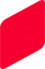Chapter 2: What is a DC-DC Converter?

Chapter 1 explained how to view the characteristics of power inductors and their different features based on their structure.
A power inductor is a functional part that consists of DC-DC converters and other voltage shifting circuits, and so their specific advantages and disadvantages and the selection of constants must be based on the operation mechanism of the DC-DC converter. This chapter describes the operation mechanism of DC-DC converters and the role of power inductors.

2.1 Overview of DC-DC converters

DC-DC converter is a collective term for circuits that convert an input voltage in a certain range to a fixed output voltage. The types of DC-DC converters are linear regulator and switching regulator. The circuit configuration also varies depending on whether the input voltage is stepped down or stepped up, and these include a wide range of types.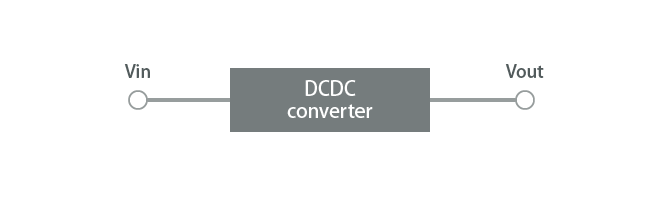Figure 2-1 Definition of DC-DC converter

2.2 Need for DC-DC converters

DC-DC converters are needed for power supply circuits only. The CPU, memory, LEDs, and other devices require many different DC voltages to run. However, in the lithium-ion batteries used in mobile devices, for example, the battery voltage (power supply) outputs about 3.7 V only. DC-DC converters are needed for adjusting these differences in voltages. DC-DC converters are required in most electronic devices, and typically, a large number of them are used in a device.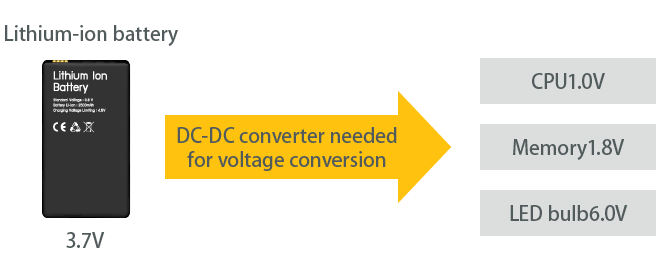Figure 2-2 Need for DC-DC converters

2.3 Classification of DC-DC converters

DC-DC converters can be classified as linear regulators or switching regulators.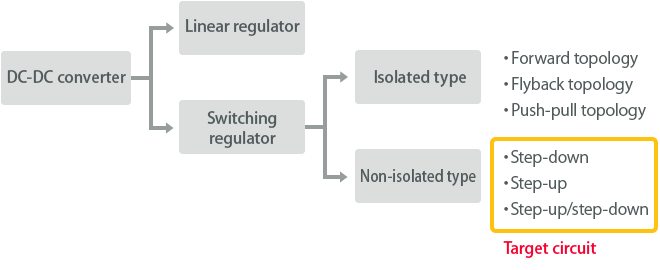Figure 2-3 Classification of DC-DC converters

2.3.1 Principles of linear regulators

A linear regulator is the simplest conversion method, and the voltage is converted by voltage division using resistors. For example, to set half of an input voltage as the output voltage, an equal load resistance and regulator variable resistance are used to divide the input voltage by one-half.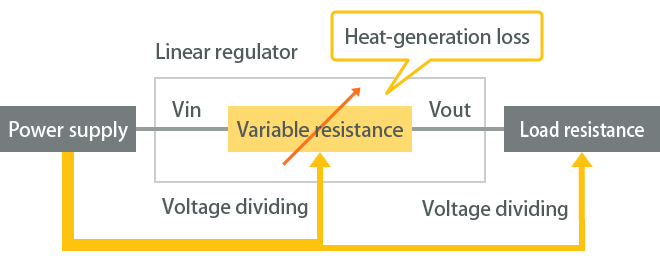Figure 2-3-1 Principles of linear regulators

While this method is simple and low-cost, one drawback is that it uses resistors, and larger potential differences for the input/output result in higher power losses (lower efficiency). Consequently, this reduces the battery runtime for mobile devices. Furthermore, a cooling mechanism is sometimes installed for the purpose of reducing the heat generated by the power losses. For this reason, linear regulators are commonly used when the power used is small or when the input/output potential difference is small. Switching regulators (explained next) are used in most circuits with a relatively higher power usage.

2.3.2 Principles of switching regulators

The switching regulator consists of a circuit combining switching elements, inductors, capacitors, and other functional parts. The switch is turned ON or OFF at high speed to enable adjustment of the output voltage, and ideally, the voltage is converted without any power loss.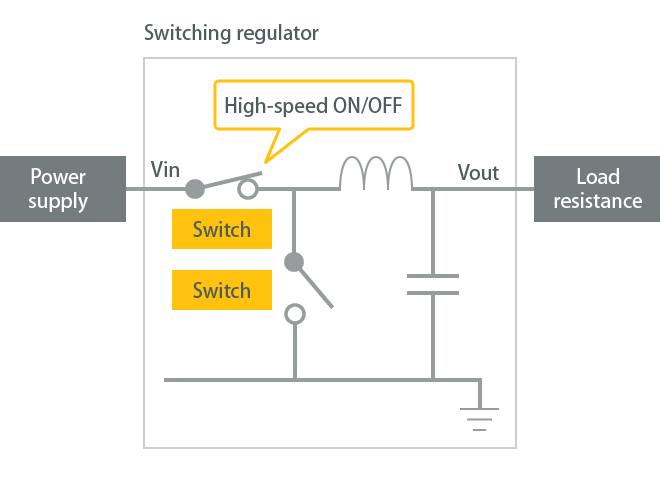Figure 2-3-2 Principles of switching regulators

This method is further divided into isolated and non-isolated types. The isolated-type switching regulator is a method where the input voltage (primary side) and output voltage (secondary side) are isolated by a transformer. This is used to prevent electric shock and electric leakage when converting high-voltage circuits. In contrast to the isolated type, the non-isolated type is a method where a transformer is not used, and the input and output are not isolated. Most mobile devices and in-car devices using batteries use non-isolated DC-DC converters because of their low voltage.

Contents：Chapter 2: What is a DC-DC Converter?
 2.1 Overview of DC-DC converters 2.2 Need for DC-DC converters 2.3 Classification of DC-DC converters 2.5 Operating principles of DC-DC converters